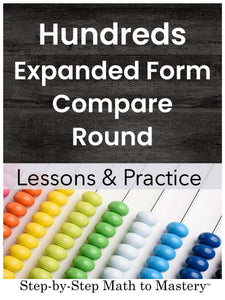# Hundreds | Expanded Form, Comparing Numbers, Rounding Off

• \$12.00
Unit price per
Shipping calculated at checkout.

## Hundreds: Expanded Form, Comparing, & Rounding Numbers

### 8 Step-by-Step Math Lessons + Practice | Special Education Math Intervention | Second Grade Math Level

This Hundreds: Expanded Form, Comparing Numbers, and Rounding Off lesson and practice workbook includes an example IEP goal, 8 step-by-step math lessons, and 50 weekly practice sheets. This evidence-based math intervention is tied to second grade standards and is great for special education math goals and tier 2 small group math interventions (RTI).

Part 1 of this workbook has 8 lessons teaching expanded form, comparing and rounding off three-digit numbers.

Part 2 is a collection of 50 weekly practice sheets for review and skill maintenance. After completing these lessons, students can continue on to “Thousands: Expanded Form, Comparing, and Rounding”.

★ Student Booklet Cover

★ 2 Lessons on Expanded Form

★ 2 Lessons on Comparing

★ 4 Lessons on Rounding Off

★ 50 Weekly Practice Sheets

152 Pages

## Students Will Be Able To:

• Identify place value positions. Be able to answer, “What digit is in the tens place?”
• Write numbers in expanded form with base ten block visuals.
• Write numbers in expanded form without any visuals.
• Compare two-digit numbers.
• Compare three-digit numbers.
• Round two-digit numbers to the nearest ten.
• Round three-digit numbers to the nearest ten.
• Round three-digit numbers to the nearest ten, up from 9 in the ones place.
• Round three-digit numbers to the nearest hundred.

### Lessons Are Tied to These Second Grade Math Standards:

• Read and write numbers to 1000 using base-ten numerals, number names, and expanded form. (CCSS 2.NBT.A.3)

• Compare two three-digit numbers based on meanings of the hundreds, tens, and ones digits, using >, =, and < symbols to record the results of comparisons. (CCSS 2.NBT.A.4)

### Not sure where to start?

Watch a video overview of Step-by-Step Math to Mastery or read the transcript here.

### Step-by-Step Math to Mastery™ Resources:

Make math easier to understand.

• Help students over math hurdles with clear, sequential, scaffolded lessons.
• Prevent overwhelm. Build student confidence.

Make math easier to teach.

• Save hours of planning and piecing together materials.
• Paraeducators can deliver quality instruction independently. Open & teach.

### Reasons You'll Love Step-by-Step Math to Mastery™ Resources

• Boosts student confidence and progress
• Time-saving: print and teach
• Easy prep: only black ink is needed
• Easy to teach, paraeducator-friendly
• Example IEP goals and shorter term objectives
• Consistent & predictable format
• Lots of practice repetitions
• Scaffolded with structured workspaces
• Fewer problems on a page, white space, minimal visual clutter
• Tied to standards
• Explicit/Direct instruction
• Systematic & sequential
• Mastery approach--teaching one topic at a time, one strategy at a time
• Lessons have "I Can" statements, model problems, guided practice, & independent practice
• Each workbook can be used individually as a stand-alone intervention for that skill or they can be used together, taking students from the basics of number sense and addition in first grade all the way to dividing fractions and decimals in fifth grade.
• Can also be used with older students (middle & high school) to help fill the gaps in their learning.